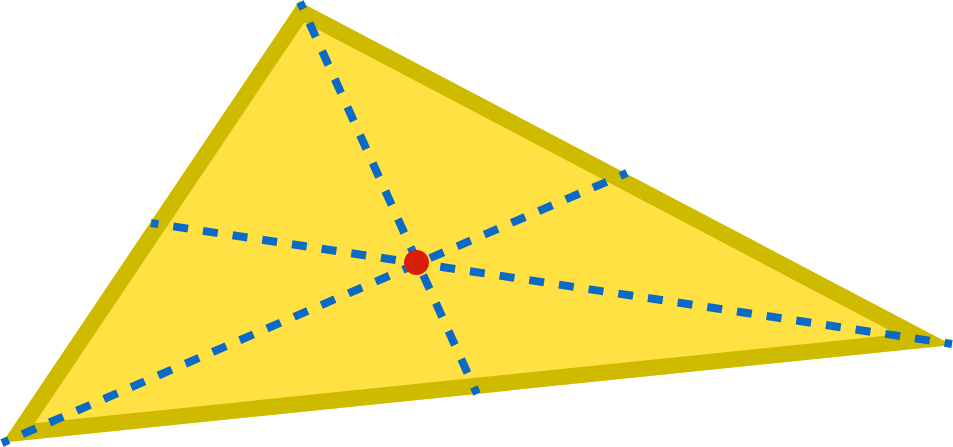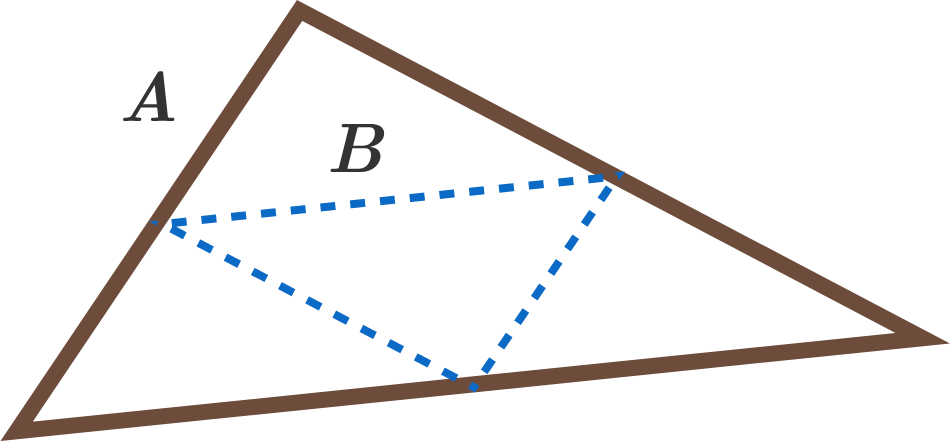# Empty Triangle!

The center of gravity of a solid triangle is the point where its medians intersect (its centroid).Consider a hollow triangle, $A,$ whose sides are made of uniformly dense, thin strings. Let $B$ be the triangle formed by connecting the midpoints of $A.$Where is the center of gravity of $A?$

For reference, here are the other three common triangle centers: circumcenter, incenter, and orthocenter.

×